• 12
•
•
•
•
•
•
•
12
Shares

# POISEULLIE’S LAW: OVERVIEW

So today in today’s article, we are going to talk about a very important and interesting concept of hydrodynamics, that is poiseullie’s laws. So going further it is necessary to understand the underlying concept of poiseullie‘s laws in the very simplest form. In poiseullie’s laws, the fluid motion should be streamlined throughout the pipe.So let’s starts

We are starting the concept with a question that in what manner a viscous fluid flows through a cylindrical pipe? In any cylindrical pipe when viscous fluid flows then they follow a convex meniscus, but why? It is because we know that a streamlined fluid has several streams of fluid.

Then some streams been near the wall of the pipe and some stream is far from the wall, In the cylindrical pipe, the longest distance from the wall is to the centre of the pipe. And we know that the pipe is cylindrical then the shape of water which are flowing through it, must be in the shape of a cylinder.
Then we can conclude that every fluid lamina in the pipe is in the shape of a cylinder. There are infinite laminae between the centre of the pipe and its wall. And as fluid starts flowing through the pipe then friction also create between the wall of the pipe and the lamina which is nearer to it. And this friction opposes the velocity of the fluid, and at the wall velocity of the fluid is taken as zero, because at this point maximum friction is applied.
Due to this friction opposing forces generated and this force is termed as laminar force or viscous force. And in the pipe each lamina opposes the velocity of others lamina, the first lamina opposes the second lamina, and the second opposes the third and so on.
And this opposition started from the wall, so the stream which is nearer to the wall has minimum velocity, and as distance increases from the wall then the velocity of the fluid also increases, and become maximum at the centre, it means its velocity is affected by the change of radius r and same occurred from the other sides of the wall, so this form the convex meniscus of the fluid in the pipe. Hope you understood.

And this is not only for the cylindrical body, this laminar flow held in all types of the body in which fluid is flowing. This gives a convex form as given below.## POISEULLIE’S LAW: BASICS

First of all, we have to know that poiseullie’s laws measure what? Poiseullie’s laws measure only the rate of flow of volume of fluid per second in the pipe. Going further let’s give a sight at what factors can volume rate depends on.

If we think little then we observe that flow rate depends directly on the pressure difference across the pipe, because if the pressure difference is small the flow of fluid become slow, but if the pressure difference is large then the flow rate increases, we have all seen it in daily life.
The second is, it depends directly on the radius of the pipe, how? Think that what would happen if radius increases, if radius increases then its cross-sectional area also increases, if its cross-sectional area increases then fluid get more space to travel and suffer a little friction, if friction is small then the flow rate increases automatically.
It is also a daily basis phenomenon, if any road is narrow then it suffers high traffic, but a broader road is free from traffic. The same is here. And third is flow rate is inversely proportional to the viscosity, larger viscosity larger friction then larger opposition and then smaller velocity than smaller flow rate. And fourth is it is inversely proportional to the length of the pipe, how? If length increases then the time of friction to the wall also increases, and friction increases then same as above.
So now we are going to derive a formula for the rate of flow of fluid volume per second.

## DERIVATION FOR POISEULLIE’S FORMULA

Let’s take a cylinder of radius R and length L as shown below,
And flowing fluid of viscosity η, and dr is the small distance between two cylindrical laminae. For understanding see the diagram below.When a fluid is in the pipe then the force of viscosity generated due to the friction between the two lamina is given as below: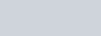Where A is the curved surface of the lamina with radius r and length L that is;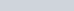And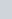is the velocity gradient, which means velocity changes with change in radius.
Here sign is negative because this force opposes the velocity of the fluid.
Then put all the values F become-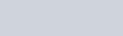And we know that fluid is flowing through the pipe due to pressure difference-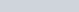This pressure can be expressed in external force per unit area, then force is given as;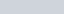In equilibrium condition, when external force became equal to the laminar frictional force that is viscous force. Equating both forces we get- Here we take only the magnitude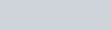After simplification we get-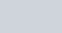Then,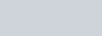. Now integrating both sides from r to R, we get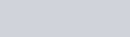On solving we get-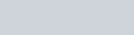Now applying the equation of continuity, we get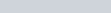, Where V is volume.

Then for a small cross-sectional area dA, we get a small volume of fluid that is dV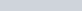Putting all values, we get-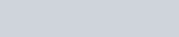On solving we get-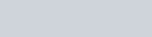Again integrating both sides from 0 to R because we want to find the whole volume of fluid in the pipe. Then we get-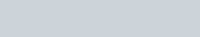On solving we get-On solving we get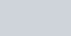This is the fluid that is flowing per second through the pipe. Watch this video for more reference.